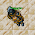## Wednesday, June 16, 2021

### TibiaGoals Riddles E9R06

Comment down below!

1.2.Nehkz
Relania

3.Nehkz
Relania

4.Nehkz
Relania

5.Hoi! My answer is 28. :) Thanks!

6.The answer is 28 because crown is 7, soft boots is 12 (but the final equation is half of the pair so 6), and golden helmet is 15 so

7.7+15+6=28

Xarkost Eilia

8.9.Golden Helmet = 15
Soft Boots = 12 (One Soft boot = 6)
Crown = 7

7 + 15 + 6 = 28

Char: Dyaniixz

10.Pinguzz

11.7+15+6=28
Ray Jek

12.28
Mian Stone'arrow

13.14.28

Skyelie Hasulhoff <3

15.16.Neo

Golden helmet = 15
Pair of soft boots = 12
Crown = 7
Single soft boot = 6
7+15+6=28

17.15+15+15=45
15+12+12=39
12-7=5
7+15+6=28..... Bebitha consentida

18.15+15+15=45
15+12+12=39
12-7=5
7+15+6=28..... Bebitha consentida

19.20.21.7+15+6= 28
By Aurora Ventresca

22.23.Nehkz
Relania

I have another answer, the previous was wrong :D It is:
7+15+6=28

24.Nehkz
Relania

I have another answer, the previous was wrong :D It is:
7+15+6=28

25.Nehkz
Relania

I have another answer, the previous was wrong :D It is:
7+15+6=28

26.28
Shizurie

27.28 Lord Neganek

28.28 shizurie

29.30.Char name: Fyori

31.character name: pablo reformed

32.28 /Norelli

33.28
Character: Amy Meow

34.15 + 15 + 15 =45
15 + 12 + 12 =39
12 - 7 = 5
7 + 15 + 6 = 28

Toxic Hasulhoff

35.31

pameliin

36.Golden helmet= 15
Soft boots= 12
Crown= 7
15+12+7=34
Eternal hunger
Antica

37.Golden helmet= 15
Soft boots= 12
Crown= 7
15+12+7=34
Eternal hunger
Antica

38.Golden helmet= 15
Soft boots= 12
Crown= 7
15+12+7=34
Eternal hunger
Antica

39.28

Lovely olka

40.R=28
samuray de carro :)

41.28 Demonovsky

42.